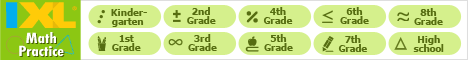Java Games: Flashcards, matching, concentration, and word search.# Geometric Terms

Match the term with the correct definition.

AB
right angleA 90 degree angle.
equilateral triangleA triangle with all sides equal.
scalene triangle A triangle having three unequal sides and angles.
vertexThe intersection point of two sides.
right triangleA triangle with one internal angle equal to 90 degrees.
pentagonA polygon with 5 sides and 5 angles.
squareA rectangle having all four sides of equal length.
intersecting linesLines that cross each other.
perpendicular linesTwo lines that cross each other to form a 90 degree angle.
acute angleAn angle less than 90 degrees.
chordThe line segment between two points on a given curve.
radiusA straight line extending from the center of a circle to the surface.
line segmentOne part of a line.
LineA continuous extent of length.
pointA position in space.
rectangleA quadrilateral with opposite sides parallel.
circleA closed plane.
closed curveA curve that is continuous with meeting end points.
hexagonA six sided figure
obtuse angleAn angle greater than 90 degrees.Marty Pettigrew

 This activity was created by a Quia Web subscriber. Learn more about QuiaCreate your own activities